### Sources

#### R packages

`"ape"` (Paradis et al 2004)

`"geiger"` (Harmon et al 2008)

#### Data

IMI data for primates (`"e.dataIMI_corrected.csv"`), Log10 Values of IMI and mass for 118 primate species.

Primate Phylogenies (`"e.IMI.TreeBlock_10kTrees_Primates_Version3.nex"`), 100 primate phylogenies sampled from 10kTrees and trimmed to species in data set.

### Codes

#### Setup

Open libraries, import and check data, set variables.

```library(ape)
library(geiger)

source(".../BayesModelS_v22.R")  #provide a path for the location of the source file that includes the functions

factorName = c() #enter variable names here that should be treated as factors
missingList = c("Homo_sapiens") #Species listed here are exluded from model fitting, and values for response are predicted

pathO = "./"
colnames(data)
```
```##  "Species"            "IMILog10"           "MassLog10"
```
```formula = "IMILog10 ~ MassLog10"
```

#### Analysis

Select and check Bayesian linear model, run analysis, and predict value of unknown tip.
Input for blm function:
formula, data, treeDataAll, factorName, missingList: assigned in previous code
currentValue: sets the starting point for MCMC, default is 0. To specify a value, a list of variables should be provided
nposterior: the number of posterior draws you want. For the sake of time, this value was set to 20100 for this online example. Actual analysis performed 200100 draws
burnin, thin: the burnin rate and thin rate in the MCMC analysis. Both set to 100 for this example.
varSelection: estimates branch length scaling parameters lambda or kappa or both. Can have three values: "random" means nothing is specified and a model selection process for lambda or kappa is conducted (while simultaneously estimating the parameter selected), while "lambda" or "kappa" estimates only their respective parameters in the MCMC analysis.
lambdaUpperBound: default to 1.
kappaUpperBound: default to 1.
lambdaValue, kappaValue: this enables the user to fix the value of lambda or kappa to use in the analysis. If a value is specified, then MCMC uses this value during the MCMC analysis, rather than estimating it.
restriction: default to "no restriction". This would control whether the user wants to manually have one variable always included in the analysis, specified by name.
path: the output folder.

```bmselection = blm(formula, data, treeDataAll, factorName = factorName, missingList = missingList, currentValue = 0, nposterior = 20100, burnin = 100, thin = 100, varSelection = "lambda", lambdaValue = NA, kappaValue = NA, path = pathO)
```
```##  "Those species are deleted from regression"
##  "Homo_sapiens"
##  "Those species are in missingList"
##  "Homo_sapiens"
##  "pre-analysis begins..."
##  "pre-analysis finished, Bayesian posterior draw begins..."
##  "regression finished 25%"
##  "regression finished 50%"
##  "regression finished 75%"
##  "regression finished 100%"
##  "Bayesian posterior draw finished, writing files..."
##  "posterior sample is written in the file ./ result.csv"
##  "dataset is written in the file ./ data.csv"
##  "files writing completed..."
```
```modelChecking(bmselection, missingList, pathO)
```
```##  "initialize analysis, predictive draw begins..."
##  "predictive draw finished, model checking begins..."
```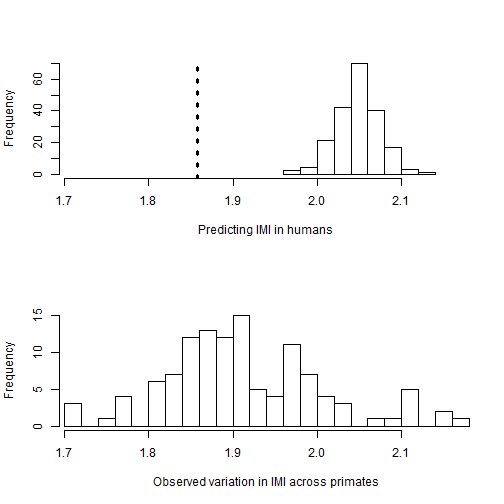```##  "model checking result finished, written in file ./ modelChecking.pdf"
```
```analysis(bmselection, path = pathO)
```
```##  "initialize analysis and get posterior sample ends, analysis begins..."
##  "model indicator written in file ./ modelIndicator.csv"
##  "initial analysis completed, plot the results..."
```
```##  "plot ploted in the file ./ outputBayesianModel.pdf"
##  "plot completed, analysis finished..."
```
```predict(bmselection, missingList, path = pathO) #this predicts response in "missingList" species
```
```##  "initialize analysis, predictive draw begins..."
##  "predictive draw end, begin for missing data analysis"
```
```##  "missing data analysis end, begin printing out the result"
```
```##                min 2.5%q 25%q median  mean  75%q 97.5%q   max
## Homo_sapiens 1.969 1.995 2.03  2.049 2.049 2.068  2.108 2.122
```

#### Display results

After importing the results from analysis, any number of methods can be used to display them. This example includes several possibilities

```outputPosterior = read.csv("./ result.csv")

# example output for posterior sample
plot(outputPosterior\$lkhoodSample, xlab="Iteration", ylab="Likelihood", cex=0.8, pch=1)
```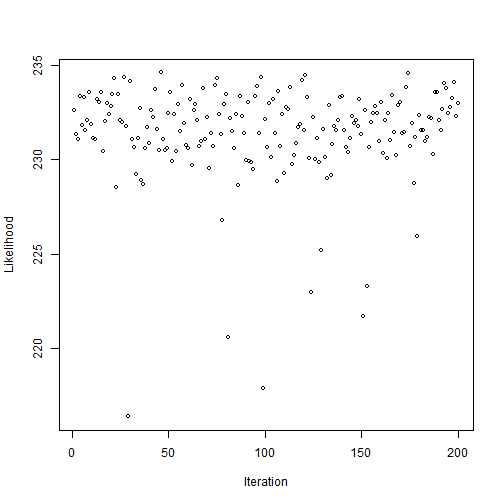```hist(outputPosterior\$lkhoodSample, n=25, xlab="Likelihood", main="")
```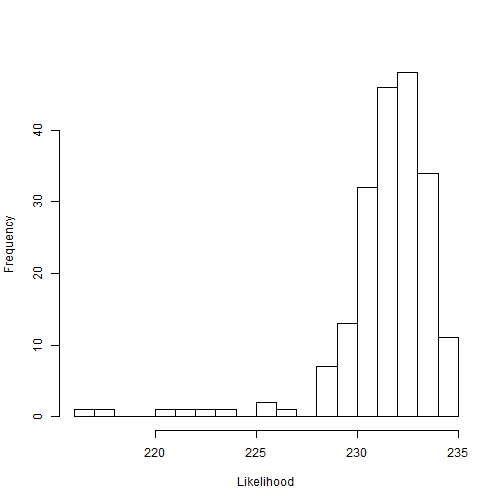```hist(outputPosterior\$coef.MassLog10, xlab="Regression Coefficient", main="", n=25)
```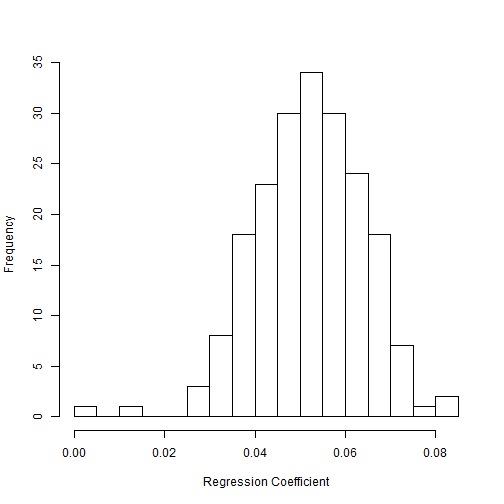```sum(outputPosterior\$MassLog10)
```
```##  199
```
```mean(subset(outputPosterior\$coef.MassLog10,abs(outputPosterior\$coef.MassLog10)>0) ) #mean coefficient
```
```##  0.05232
```
```hist(outputPosterior\$lambdaSample, n=20, xlab="lambda", main="")
```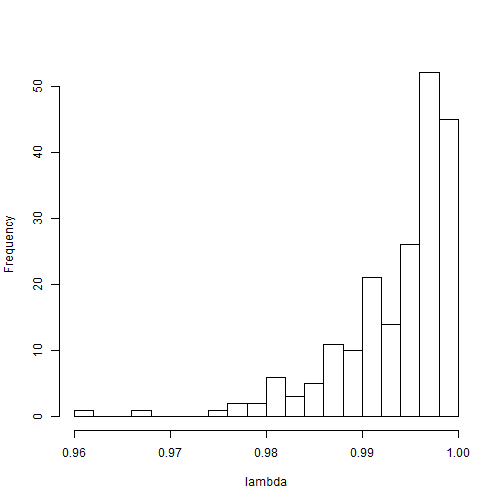```mean(outputPosterior\$lambda)
```
```##  0.9936
```
```outputPosteriorHsapeiens = read.csv("./predictions.csv")

#example output for prediction distribution of value on missing tip
par(mfrow=c(2,1))
hist(outputPosteriorHsapeiens\$Homo_sapiens, xlab="Predicting IMI in humans", main="", xlim=c(min(data[,2], na.rm=TRUE), max(data[,2], na.rm=TRUE)))
abline(v= data[51,2], col=1, lwd=3, lty=3)

hist(data[,2], xlab="Observed variation in IMI across primates", main="", xlim=c(min(data[,2], na.rm=TRUE), max(data[,2], na.rm=TRUE)), n=20)
```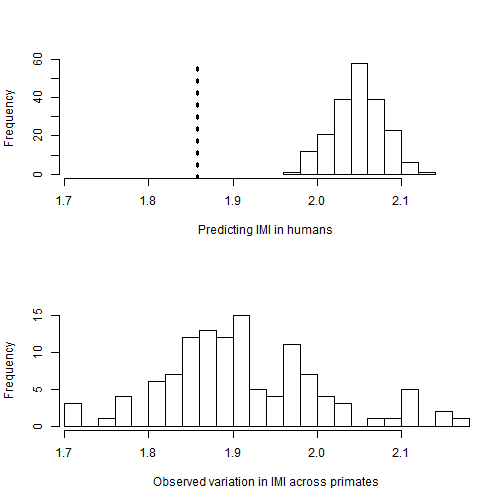### References

• Paradis E., Claude J. & Strimmer K. 2004. APE: analyses of phylogenetics and evolution in R language. Bioinformatics 20: 289-290.
• Harmon Luke J, Jason T Weir, Chad D Brock, Richard E Glor, and Wendell Challenger. 2008. GEIGER: investigating evolutionary radiations. Bioinformatics 24:129-131.
• David Orme, Rob Freckleton, Gavin Thomas, Thomas Petzoldt, Susanne Fritz, Nick Isaac and Will Pearse (2013). caper: Comparative Analyses of Phylogenetics and Evolution in R. R package version 0.5.2. http://CRAN.R-project.org/package=caper
• Arnold, C., L. J. Matthews, and C. L. Nunn. 2010. The 10kTrees Website: A New Online Resource for Primate Phylogeny. Evolutionary Anthropology 19:114-118